# IMO Class 3 level 2 Question Paper - Set 5

Check these Class 3 IMO Level 2 questions from previous years Maths Olympiad exams.

For our paid members, we offer comprehensive benefits, including access to detailed explanations for all questions in our Chapterwise Test Series and Mock Test Series. Our paid members have the opportunity to attempt more than a dozen tests for each chapter covered in the SOF IMO syllabus for Class 3. They can attempt each test multiple times to enhance their learning experience.Our courses can be bought at our BUY COURSES section.

Our Mock Tests for Class 3 IMO follow the exact pattern of SOF IMO in terms of questions, marking and time alloted for completion. This helps the students to experience of the real time exam environment and assess their exam preparedness. Since we follow the same marking system , the scores obtained at the end of each test will help identify students' weaknesses and assist them in improving.

Olympiadtester has displayed a few Chapterwise tests and Mock tests in the FREE SECTION.

1) How many angles are there inside this figure?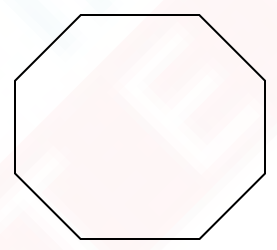OPTIONS

1. 16
2. 10
3. 8
4. 6

2) Which of the following pairs of lines are parallel?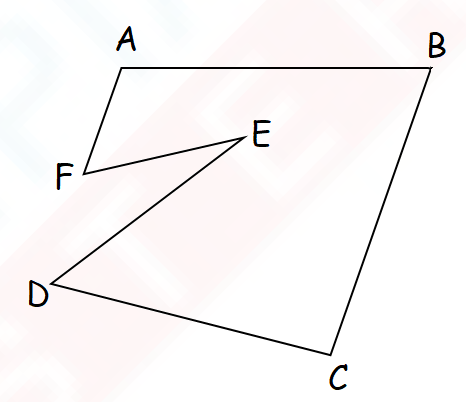OPTIONS

1.  AF and BC
2. AB and FE
3. DE and CB
4. DC and FE

3) The figure below is made up of 5 identical rectangles. Find the perimeter of the figure.OPTIONS

1. 160 cm
2. 320 cm
3. 600 cm
4. 800 cm

4) How many right angles are there inside the figure below?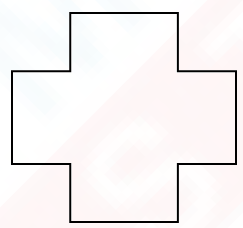OPTIONS

1. 16
2. 12
3. 8
4. 4

5) Which one of the figures below has the same area as the given figure ?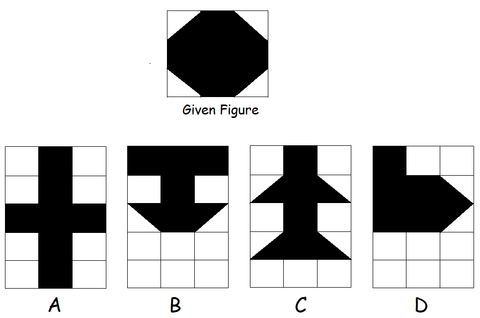OPTIONS

1. A
2. B
3. C
4. D

6) Name the pair of perpendicular lines in the figure below.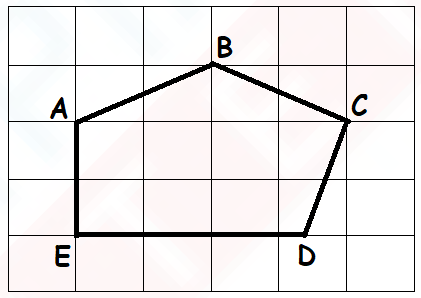1. AB ⊥ BC
2. CB ⊥ DE
3. AE ⊥ ED
4. AB ⊥ CD

7 Which figure below has more than 1 pair of parallel lines?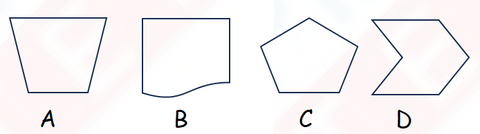OPTIONS

1. A
2. B
3. C
4. D

8) Name the pair of perpendicular lines in the figure below.OPTIONS

1. AB ⊥ BC
2. CB ⊥ DE
3. AE ⊥ ED
4. AB ⊥ CD

9) Fifteen rupees and two paises can be written as ______________________.

OPTIONS

1. ₹15.02
2. ₹15.20
3. ₹50.02
4. ₹50.20

10) Mother bought 24 oranges. How much did she pay?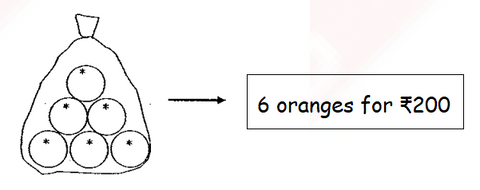OPTIONS

1. ₹400
2. ₹600
3. ₹800
4. ₹1000

11) The figure is made up of equal squares. Each square has a side of 3 cm. Find the area of the shaded part.12) The figure below is not drawn to scale. The figure is made up of two rectangles. The smaller rectangle has a length of 6 m and a breadth of 4 m. The bigger rectangle has a length of 15 m and a breadth of 8 m. Find the area of the unshaded part.13) Arrange the angles from the smallest to the greatest.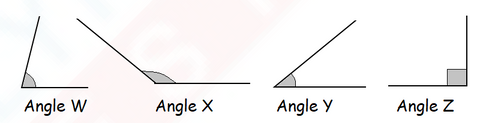Answer : _______, ________, ________, _________

14) The picture below is made up of 5 identical rectangular tiles.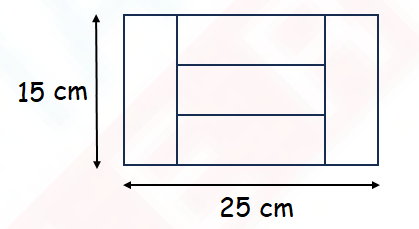a) What is the area of 1 tile?

b) What is the perimeter of 1 tile?

15) 4 identical squares were cut off from the corners of a piece of paper as shown below. Find the perimeter of the remaining piece of paper which is shaded.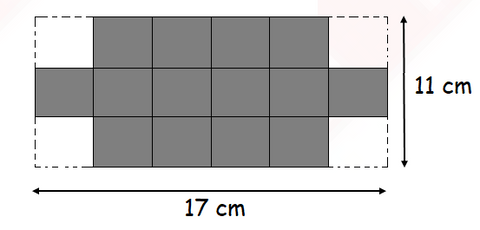Olympiadtester has displayed a few Chapterwise tests and Mock tests in the FREE SECTION.